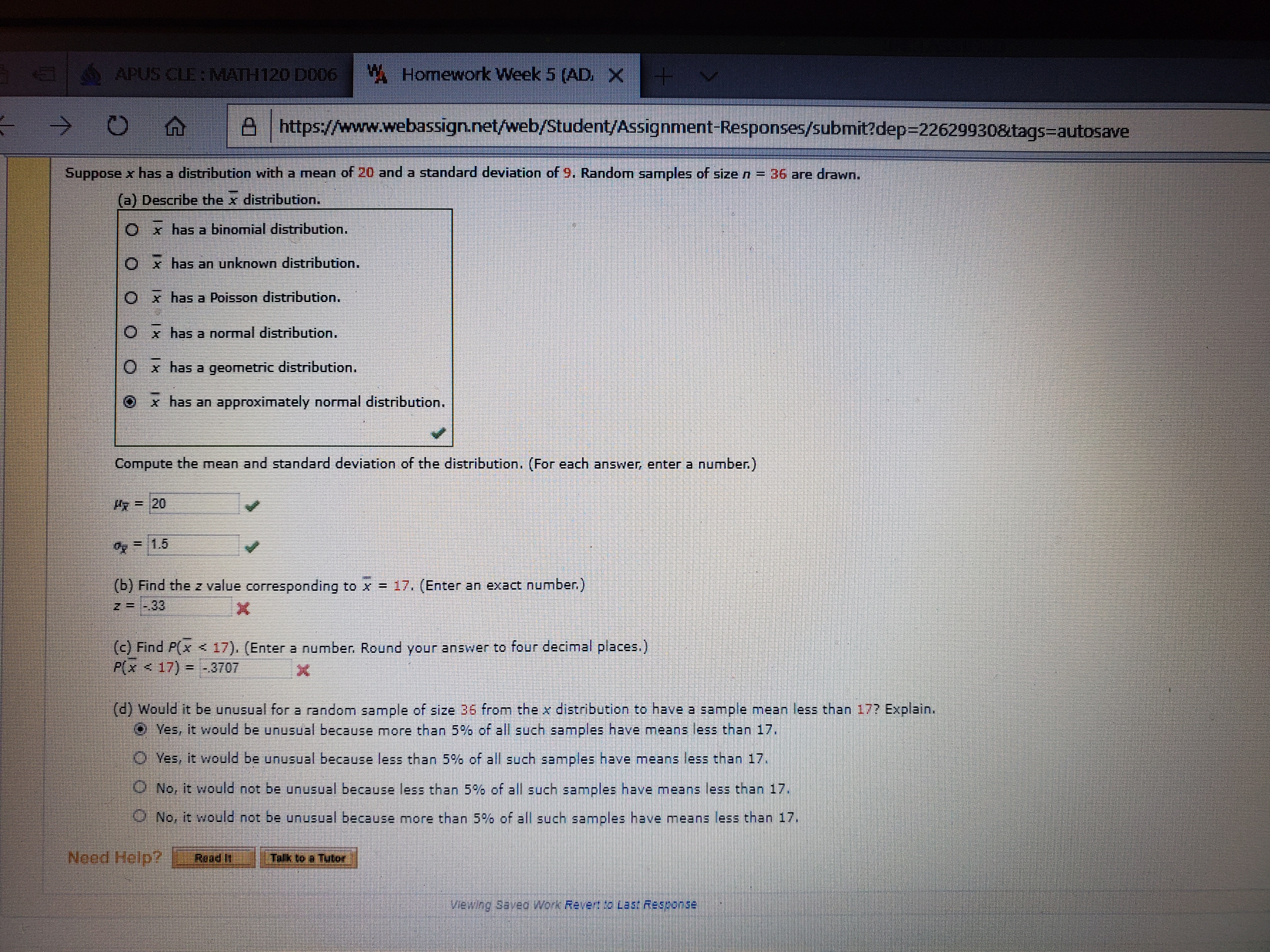# 1W Homework Week 5 (ADAPUS CLE:MATH120 D006XA https://www.webassign.net/web/Student/Assignment-Responses/submit?dep=D226299308&tags=autosave->Suppose x has a distribution with a mean of 20 and a standard deviation of 9. Random samples of size n = 36 are drawn.(a) Describe the x distribution.O x has a binomial distribution.Ox has an unknown distribution.Ox has a Poisson distribution.Ox has a normal distribution.Ox has a geometric distribution.x has an approximately normal distribution.Compute the mean and standard deviation of the distribution. (For each answer, enter a number.)收= 20O=1.5(b) Find the z value corresponding to x = 17. (Enter an exact number)z=-33(c) Find P(x < 17). (Enter a number. Round your answer to four decimal places.)P(x

Question
1 views

I'm having trouble finding probabilities and z values.help_outlineImage Transcriptionclose1 W Homework Week 5 (AD APUS CLE:MATH120 D006 X A https://www.webassign.net/web/Student/Assignment-Responses/submit?dep=D226299308&tags=autosave -> Suppose x has a distribution with a mean of 20 and a standard deviation of 9. Random samples of size n = 36 are drawn. (a) Describe the x distribution. O x has a binomial distribution. Ox has an unknown distribution. Ox has a Poisson distribution. Ox has a normal distribution. Ox has a geometric distribution. x has an approximately normal distribution. Compute the mean and standard deviation of the distribution. (For each answer, enter a number.) 收= 20 O=1.5 (b) Find the z value corresponding to x = 17. (Enter an exact number) z=-33 (c) Find P(x < 17). (Enter a number. Round your answer to four decimal places.) P(x <17) = -3707 (d) Would it be unusual for a random sample of size 36 from the x distribution to have a sample mean less than 17? Explain. O Yes, it would be unusual because more than 5% of all such samples have means less than 17. O Yes, it would be unusual because less than 5% of all such samples have means less than 17. O No, it would not be unusual because less than 5% of all such samples have means less than 17. O No, it would not be unusual because more than 5% of all such samples have means less than 17. Need Help? Talk to a Tulor Read Ii vewing Saved Work Ravert o Last Response fullscreen
check_circle

Step 1

Given info:

The mean and standard deviation of the distribution are, µ=20 and σ=26 respectively.

Step 2

(b) Computation of z value corresponding to =17:

The z value corresponding to =17 is obtained as −2, from the calculations given below:

Step 3

(c) Computation of P(<17):

The value of P(<17) is obtained as 0.0228 (rounded to four decimal places),...

### Want to see the full answer?

See Solution

#### Want to see this answer and more?

Solutions are written by subject experts who are available 24/7. Questions are typically answered within 1 hour.*

See Solution
*Response times may vary by subject and question.
Tagged in

### Other